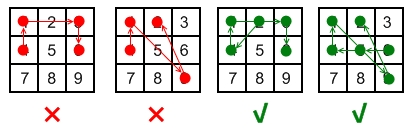351. Android Unlock Patterns

Android devices have a special lock screen with a `3 x 3` grid of dots. Users can set an "unlock pattern" by connecting the dots in a specific sequence, forming a series of joined line segments where each segment's endpoints are two consecutive dots in the sequence. A sequence of `k` dots is a valid unlock pattern if both of the following are true:

• All the dots in the sequence are distinct.
• If the line segment connecting two consecutive dots in the sequence passes through any other dot, the other dot must have previously appeared in the sequence. No jumps through non-selected dots are allowed.

Here are some example valid and invalid unlock patterns:• The 1st pattern `[4,1,3,6]` is invalid because the line connecting dots `1` and `3` pass through dot `2`, but dot `2` did not previously appear in the sequence.
• The 2nd pattern `[4,1,9,2]` is invalid because the line connecting dots `1` and `9` pass through dot `5`, but dot `5` did not previously appear in the sequence.
• The 3rd pattern `[2,4,1,3,6]` is valid because it follows the conditions. The line connecting dots `1` and `3` meets the condition because dot `2` previously appeared in the sequence.
• The 4th pattern `[6,5,4,1,9,2]` is valid because it follows the conditions. The line connecting dots `1` and `9` meets the condition because dot `5` previously appeared in the sequence.

Given two integers `m` and `n`, return the number of unique and valid unlock patterns of the Android grid lock screen that consist of at least `m` keys and at most `n` keys.

Two unlock patterns are considered unique if there is a dot in one sequence that is not in the other, or the order of the dots is different.

Example 1:

```Input: m = 1, n = 1
Output: 9
```

Example 2:

```Input: m = 1, n = 2
Output: 65
```

Constraints:

• `1 <= m, n <= 9`

351. Android Unlock Patterns
``````struct Solution;

impl Solution {
fn number_of_patterns(m: i32, n: i32) -> i32 {
let mut res = 0;
let mut visited = vec![vec![false; 3]; 3];
Self::dfs(0, None, &mut visited, &mut res, m as usize, n as usize);
res as i32
}

fn dfs(
start: usize,
prev: Option<(usize, usize)>,
visited: &mut Vec<Vec<bool>>,
all: &mut usize,
m: usize,
n: usize,
) {
if start >= m {
*all += 1;
}
if start == n {
return;
}
if let Some((r, c)) = prev {
for i in 0..3 {
for j in 0..3 {
if !visited[i][j] {
if ((i == r && j + c == 2)
|| (j == c && i + r == 2)
|| (i + r == 2 && j + c == 2))
&& !visited[(i + r) / 2][(j + c) / 2]
{
continue;
}
visited[i][j] = true;
Self::dfs(start + 1, Some((i, j)), visited, all, m, n);
visited[i][j] = false;
}
}
}
} else {
for i in 0..3 {
for j in 0..3 {
visited[i][j] = true;
Self::dfs(start + 1, Some((i, j)), visited, all, m, n);
visited[i][j] = false;
}
}
}
}
}

#[test]
fn test() {
let m = 1;
let n = 1;
let res = 9;
assert_eq!(Solution::number_of_patterns(m, n), res);
let m = 1;
let n = 2;
let res = 65;
assert_eq!(Solution::number_of_patterns(m, n), res);
}
``````